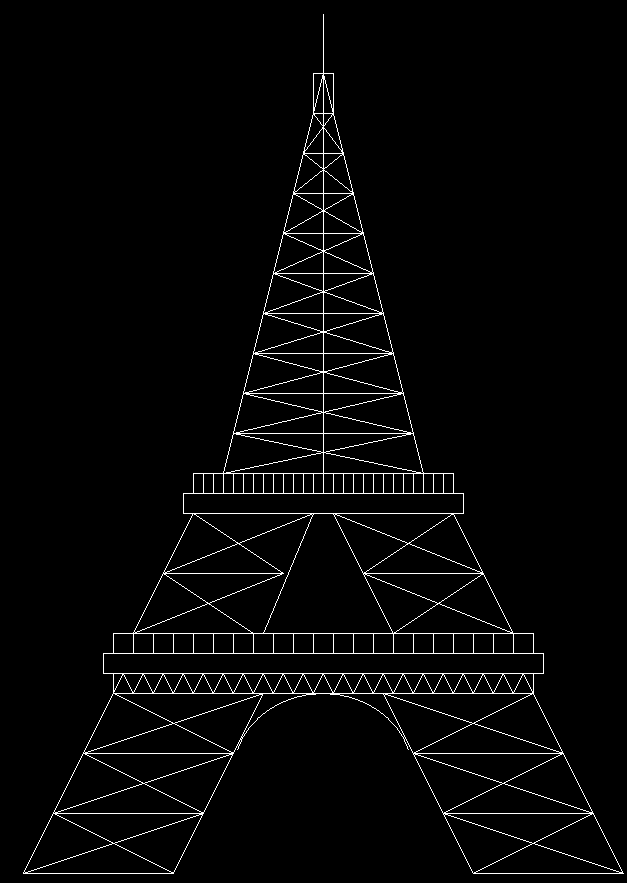# C program to draw Eiffel Tower using computer graphics

• Difficulty Level : Basic
• Last Updated : 13 Jul, 2021

In C graphics, the graphics.h functions are used to draw different shapes like circles, rectangles, etc, display text(any message) in a different format (different fonts and colors). By using graphics.h one can make programs, animations, and also games.

Function Used:

• rectangle(l, t, r, b):  A function from graphics.h header file which draws a rectangle from left(l) to right(r) & from top(t) to bottom(b).
• line(a1, b1, a2, b2):  A function from graphics.h header file which draws a line from (a1, b1) point to (a2, b2) point.
• ellipse(int x, int y, int start_angle, int end_angle, int x_radius, int y_radius): A function from graphics.h header file where x, y is the location of the ellipse. x_radius and y_radius decide the radius of the form x and y. start_angle is the starting point of the angle and end_angle is the ending point of the angle. The value of the angle can vary from 0 to 360 degrees.

Approach:

• The first step is to make the left side base of the tower. The left side base is totally built up with a line() function.
• On the left side of the base, construct a total of four lines. These lines are tiled from each other. Then join the left side of the lines with a tangent line and the right side with another tangent line. Also, do interior decoration by joining opposite sides of each base with a line. This full work has to be done by using a line() function.
• The same has to be done with the right side, similar to the one done on the left side. But the difference is that it is required to tilt bases on the opposite side.
• Then join two sides with a line() function.
• The next step is to make a half-circle by using an ellipse() function.
• Implement three rectangles by using a rectangle() function. All these rectangles will be used in decoration functions.
• Among the rectangles, one will be decorated with a continuous triangle which will be implemented by the line() function. These continuous triangle decorations will be done using a while loop.
• Another rectangle will be decorated with vertical lines which are separated by the same distance. These vertical lines are implemented by a line() function in another while loop.
• Steps followed on the lower base again have to be done here as well. The full method is totally the same here also. But here, we have implemented three bases instead of four.
• Join the two sides with a line() function.
• Implement two rectangles using the rectangle() function. Between them, the upper one is to be decorated by some vertical lines placed at the same distance from each other. These lines will be implemented by the line() function in a while loop.
• Make a while loop that will divide the height of the remaining tower and also create some decoration in it in a single while loop. This whole operation will be implemented by the line() function.
• Join the two sides with the line function. Create a rectangle using a rectangle() function on the upper side and a straight line using a line() function.

Below is the implementation of the above approach:

## C

 `// C program for the above approach``#include ``#include ``#include `` ` `// Driver Code``void` `main()``{``    ``int` `gd = DETECT, gm;`` ` `    ``// Initialize of gdriver with``    ``// DETECT macros``    ``initgraph(&gd, &gm, ``"C:\\turboc3\\bgi"``);`` ` `    ``// Declared Variables``    ``int` `a = 390, b = 390, c = 700;`` ` `    ``// Left Side``    ``// Lower Base``    ``line(300, 1000, 450, 1000);`` ` `    ``// Inside Decoration``    ``line(300, 1000, 480, 940);``    ``line(450, 1000, 330, 940);`` ` `    ``// 1st Base``    ``line(330, 940, 480, 940);`` ` `    ``// Inside Decoration``    ``line(330, 940, 510, 880);``    ``line(480, 940, 360, 880);`` ` `    ``// 2nd Base``    ``line(360, 880, 510, 880);`` ` `    ``// Inside Decoration``    ``line(360, 880, 540, 820);``    ``line(390, 820, 510, 880);`` ` `    ``// 3rd Base``    ``line(390, 820, 540, 820);`` ` `    ``// Left Tangent``    ``line(300, 1000, 390, 820);`` ` `    ``// Right Tangent``    ``line(450, 1000, 540, 820);`` ` `    ``// Joining Line``    ``line(390, 820, 810, 820);`` ` `    ``// Half Circle``    ``ellipse(600, 900, 15, 165, 90, 80);`` ` `    ``// Right Side``    ``// Lower Base``    ``line(750, 1000, 900, 1000);`` ` `    ``// Inside Decoration``    ``line(750, 1000, 870, 940);``    ``line(720, 940, 900, 1000);`` ` `    ``// 1st Base``    ``line(720, 940, 870, 940);`` ` `    ``// Inside Decoration``    ``line(720, 940, 840, 880);``    ``line(870, 940, 690, 880);`` ` `    ``// 2nd Base``    ``line(690, 880, 840, 880);`` ` `    ``// Inside Decoration``    ``line(690, 880, 810, 820);``    ``line(840, 880, 660, 820);`` ` `    ``// 3rd Base``    ``line(660, 820, 810, 820);`` ` `    ``// Left Tangent``    ``line(750, 1000, 660, 820);`` ` `    ``// Right Tangent``    ``line(900, 1000, 810, 820);`` ` `    ``// Rectangles For Decoration``    ``rectangle(390, 800, 810, 820);``    ``rectangle(380, 780, 820, 800);``    ``rectangle(390, 760, 810, 780);`` ` `    ``// Triangle Decoration``    ``while` `(a <= 790) {``        ``line(a, 820, a + 10, 800);``        ``line(a + 10, 800, a + 20, 820);``        ``a = a + 20;``    ``}`` ` `    ``// Vertical Line Decoration``    ``while` `(b <= 810) {``        ``line(b, 760, b, 780);``        ``b = b + 20;``    ``}`` ` `    ``// Left Side``    ``// Upper Base``    ``line(410, 760, 530, 760);`` ` `    ``// Inside Decoration``    ``line(410, 760, 560, 700);``    ``line(530, 760, 440, 700);`` ` `    ``// 1st Base``    ``line(440, 700, 560, 700);`` ` `    ``// Inside Decoration``    ``line(440, 700, 590, 640);``    ``line(560, 700, 470, 640);`` ` `    ``// 2nd base``    ``line(470, 640, 590, 640);`` ` `    ``// Left Tangent``    ``line(410, 760, 470, 640);`` ` `    ``// Right Tangent``    ``line(540, 760, 590, 640);`` ` `    ``// Right Side``    ``// Upper Base``    ``line(670, 760, 790, 760);`` ` `    ``// Inside Decoration``    ``line(670, 760, 760, 700);``    ``line(790, 760, 640, 700);`` ` `    ``// 1st Base``    ``line(640, 700, 760, 700);`` ` `    ``// Inside Decoration``    ``line(640, 700, 730, 640);``    ``line(760, 700, 610, 640);`` ` `    ``// 2nd Base``    ``line(610, 640, 730, 640);`` ` `    ``// Left Tangent``    ``line(670, 760, 610, 640);`` ` `    ``// Right Tangent``    ``line(790, 760, 730, 640);`` ` `    ``// Joining Line``    ``line(470, 640, 730, 640);`` ` `    ``// Rectangle For Decoration``    ``rectangle(460, 620, 740, 640);``    ``rectangle(470, 600, 730, 620);`` ` `    ``// Redeclaring Variable``    ``b = 470;`` ` `    ``// Vertical Line Decoration``    ``while` `(b <= 730) {``        ``line(b, 600, b, 620);``        ``b = b + 10;``    ``}`` ` `    ``// Redeclaring Variable``    ``a = 600;``    ``b = 500;`` ` `    ``// Middle Line``    ``line(600, 600, 600, 140);`` ` `    ``// Upper Most Decoration``    ``while` `(b >= 240) {``        ``if` `(b == c)``            ``break``;``        ``else` `{``            ``line(b, a, c, a);``            ``line(b, a, c - 10, a - 40);``            ``line(b + 10, a - 40, c, a);``            ``a = a - 40;``            ``b = b + 10;``            ``c = c - 10;``        ``}``    ``}`` ` `    ``// Tangent Lines``    ``line(500, 600, 590, 240);``    ``line(700, 600, 610, 240);``    ``rectangle(590, 200, 610, 240);`` ` `    ``// Holding The Screen For A While``    ``getch();`` ` `    ``// Close the initialized gdriver``    ``closegraph();``}`

Output:My Personal Notes arrow_drop_up